# If liabilities are \$53,000 and assets are \$173,500, then equity equals: Multiple Choice \$120,500 \$173,500 \$226,500...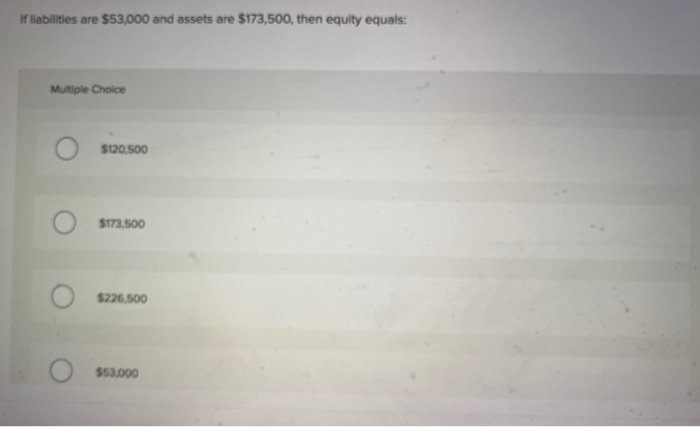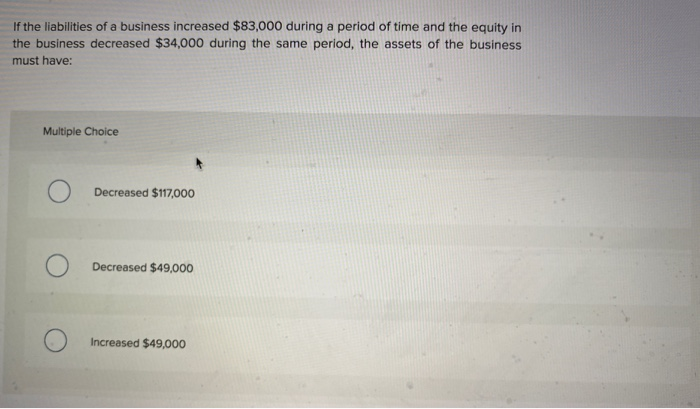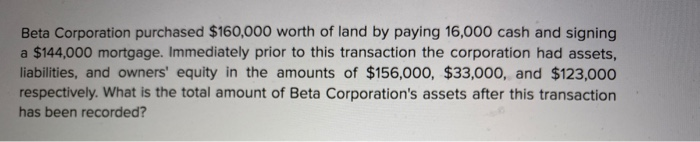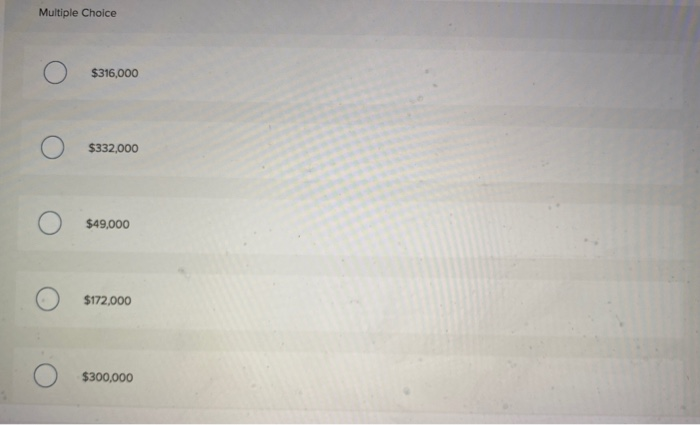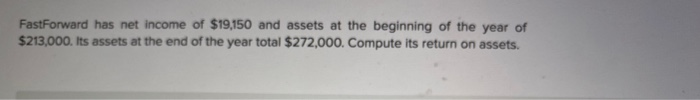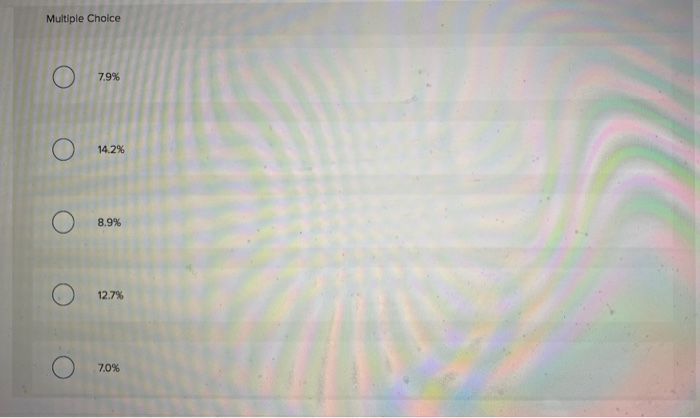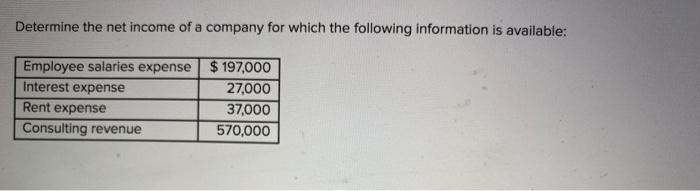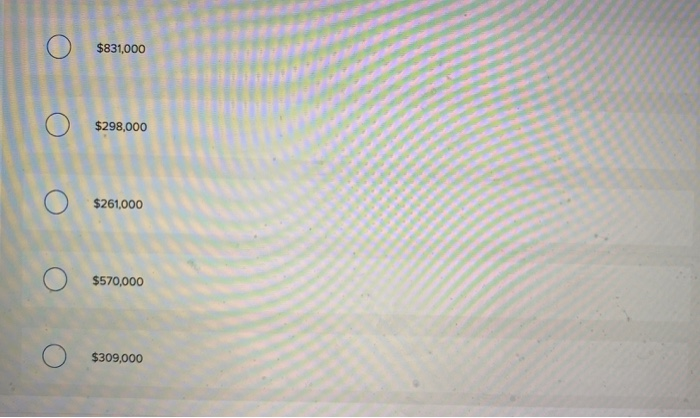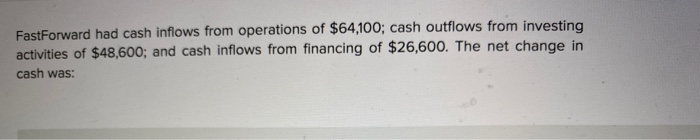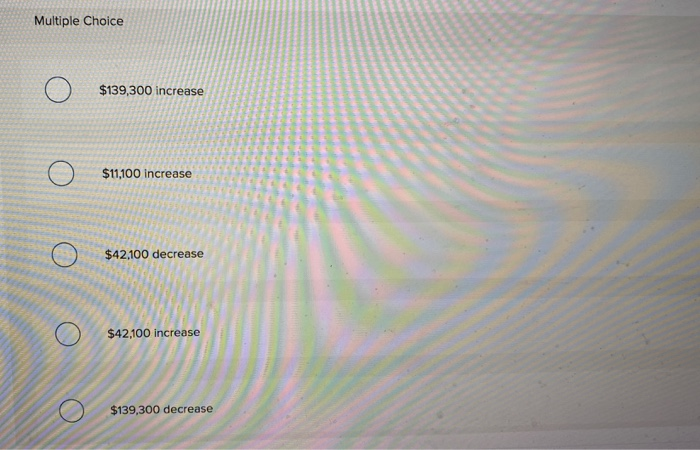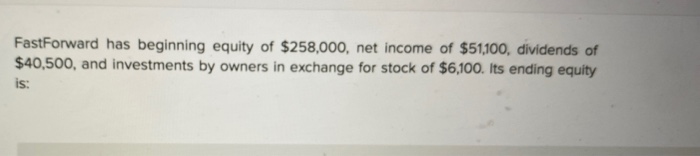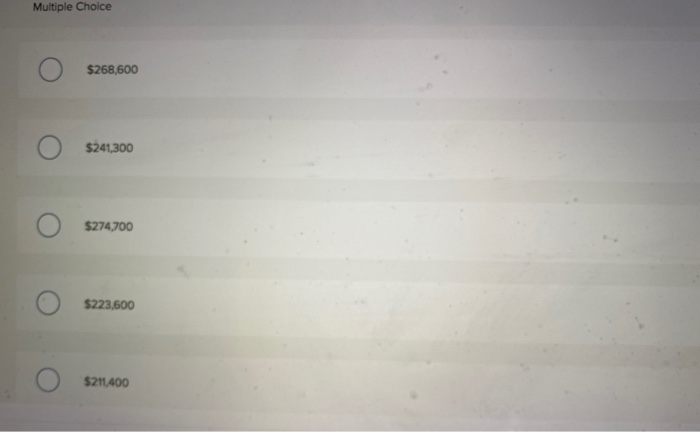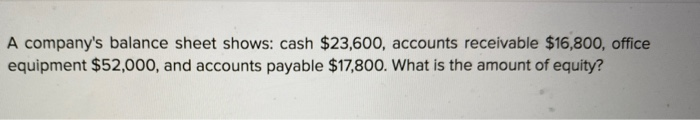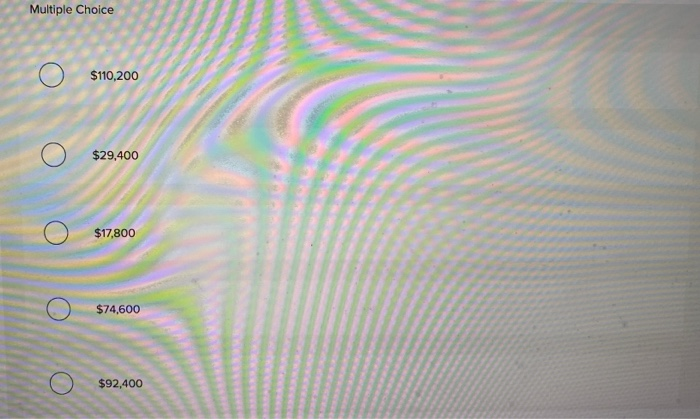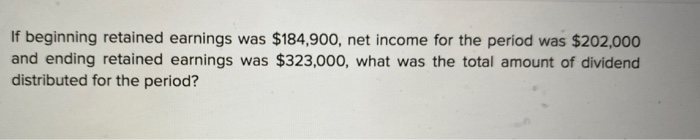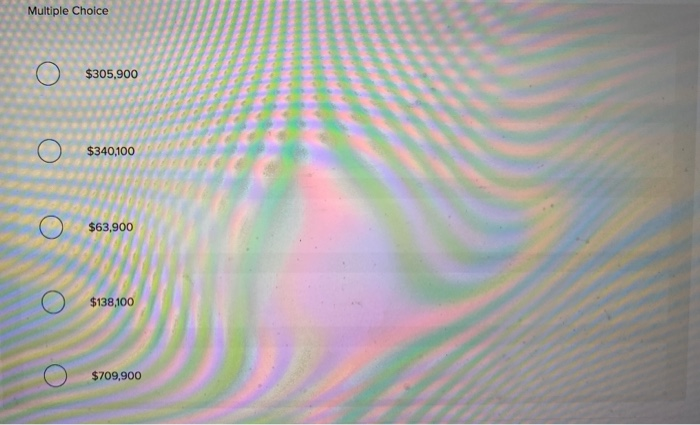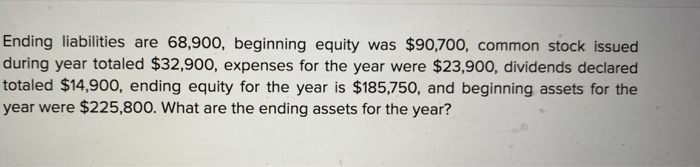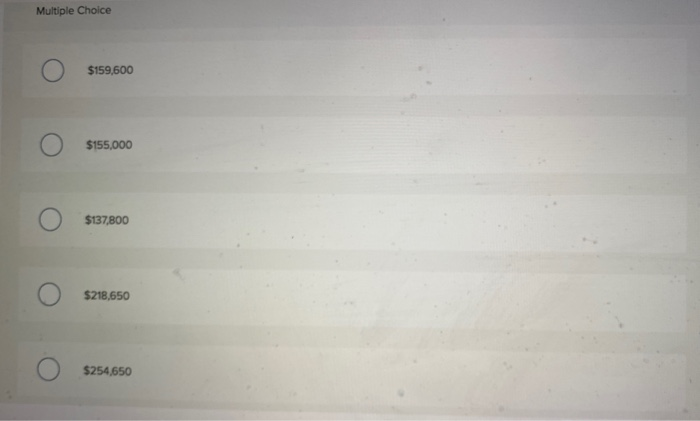If liabilities are \$53,000 and assets are \$173,500, then equity equals: Multiple Choice \$120,500 \$173,500 \$226,500 \$53,000
If the liabilities of a business increased \$83,000 during a period of time and the equity in the business decreased \$34,000 during the same period, the assets of the business must have: Multiple Choice Decreased \$117,000 Decreased \$49,000 Increased \$49,000
Beta Corporation purchased \$160,000 worth of land by paying 16,000 cash and signing a \$144,000 mortgage. Immediately prior to this transaction the corporation had assets, liabilities, and owners' equity in the amounts of \$156,000, \$33,000, and \$123,000 respectively. What is the total amount of Beta Corporation's assets after this transaction has been recorded?
Multiple Choice \$316,000 \$332,000 \$49,000 \$172,000 \$300,000
FastForward has net income of \$19,150 and assets at the beginning of the year of \$213,000. Its assets at the end of the year total \$272,000. Compute its return on assets.
Multiple Choice 7.9% 14.2% 8.9% 12.7% 7.0%
Determine the net income of a company for which the following information is available: Employee salaries expense Interest expense Rent expense Consulting revenue \$ 197,000 27,000 37,000 570,000
\$831,000 \$298,000 \$261,000 \$570,000 \$309,000
FastForward had cash inflows from operations of \$64,100; cash outflows from investing activities of \$48,600; and cash inflows from financing of \$26,600. The net change in cash was:
Multiple Choice \$139,300 increase \$11,100 increase \$42.100 decrease \$42,100 increase \$139,300 decrease
FastForward has beginning equity of \$258,000, net income of \$51100, dividends of \$40,500, and investments by owners in exchange for stock of \$6,100. Its ending equity is:
Multiple Choice \$268,600 \$241,300 \$274,700 \$223,600 \$211,400
A company's balance sheet shows: cash \$23,600, accounts receivable \$16,800, office equipment \$52,000, and accounts payable \$17,800. What is the amount of equity?
Multiple Choice \$110,200 \$29,400 \$17,800 \$74,600 \$92,400
If beginning retained earnings was \$184,900, net income for the period was \$202,000 and ending retained earnings was \$323,000, what was the total amount of dividend distributed for the period?
Multiple Choice \$305,900 \$340,100 \$63,900 \$138,100 \$709,900
Ending liabilities are 68,900, beginning equity was \$90,700, common stock issued during year totaled \$32,900, expenses for the year were \$23,900, dividends declared totaled \$14,900, ending equity for the year is \$185,750, and beginning assets for the year were \$225,800. What are the ending assets for the year?
Multiple Choice \$159,600 \$155,000 \$137,800 \$218,650 \$254,650

This Homework Help Question: "If liabilities are \$53,000 and assets are \$173,500, then equity equals: Multiple Choice \$120,500 \$173,500 \$226,500..." No answers yet.

We need 10 more requests to produce the answer to this homework help question. Share with your friends to get the answer faster!

0 /10 have requested the answer to this homework help question.

Once 10 people have made a request, the answer to this question will be available in 1-2 days.
All students who have requested the answer will be notified once they are available.
##### Add Answer to: If liabilities are \$53,000 and assets are \$173,500, then equity equals: Multiple Choice \$120,500 \$173,500 \$226,500...
Similar Homework Help Questions
• ### The statement of cash flows reports: Multiple Choice Ο Assets, liabilities, and equity. Ο Revenues, gains,...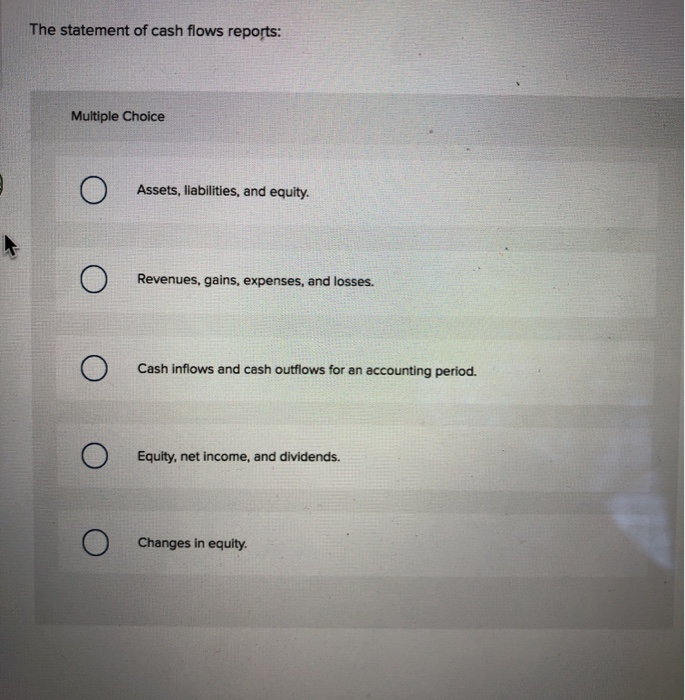The statement of cash flows reports: Multiple Choice Ο Assets, liabilities, and equity. Ο Revenues, gains, expenses, and losses. Ο Cash inflows and cash outflows for an accounting period. cash intows an Ο Equity, net income, and dividends. Ο Changes in equity.

• ### Determine the net income of a company for which the following information is available: Employee salaries...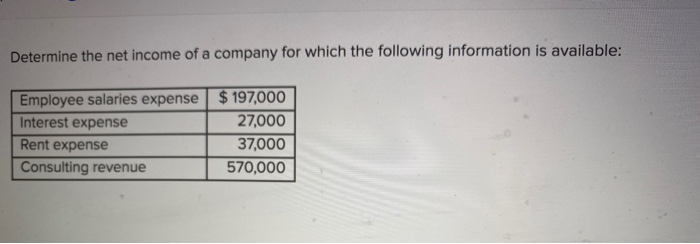Determine the net income of a company for which the following information is available: Employee salaries expense \$ 197,000 Interest expense 27,000 Rent expense 37,000 Consulting revenue 570,000 We were unable to transcribe this imageFastForward has beginning equity of \$258,000, net income of \$51,100, dividends of \$40,500, and investments by owners in exchange for stock of \$6,100. Its ending equity is: Multiple Choice О O \$268,600 O \$241,300 О O \$274,700 \$223,600 O \$211,400 A company's balance sheet shows: cash...

• ### The accounting equation is defined as: Multiple Choice Assets = Liabilities - Stockholders' Equity. Net Income...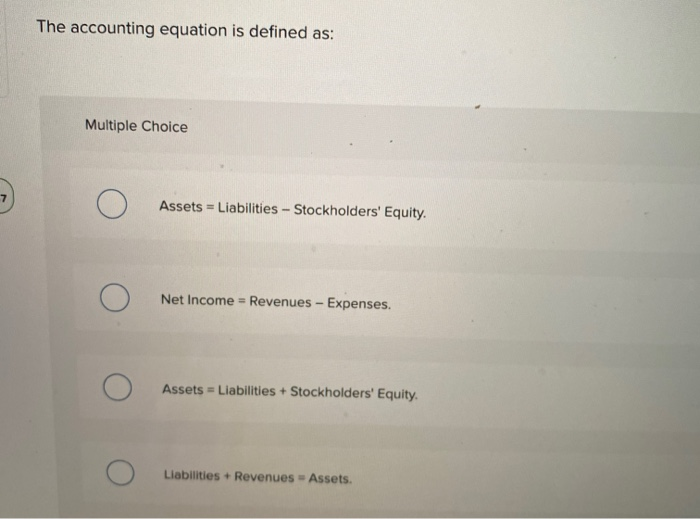The accounting equation is defined as: Multiple Choice Assets = Liabilities - Stockholders' Equity. Net Income = Revenues - Expenses. Assets = Liabilities + Stockholders' Equity. Liabilities + Revenues - Assets.

• ### Which of the following is a true statement? Multiple Choice Expenses increase owners’ equity and decrease...

Which of the following is a true statement? Multiple Choice Expenses increase owners’ equity and decrease liabilities. Revenue decreases owners’ equity and expenses increase owners’ equity. Revenue increases owners’ equity and expenses decrease owners’ equity. Revenue decreases owners’ equity and increases liabilities. Which of the following statements is not true regarding the adoption of ASC Topic 606 guidance for revenue recognition? Multiple Choice When using the cumulative approach, the prior three years of financial statements need to be restated. Under...

• ### A company's assets totaled \$24,350 and liabilities totaled \$8,510 at the beginning of the year. During...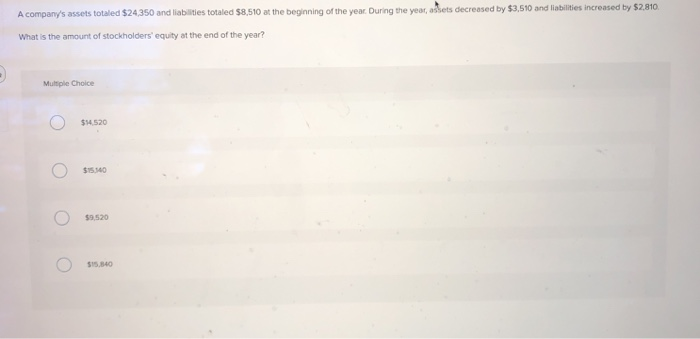A company's assets totaled \$24,350 and liabilities totaled \$8,510 at the beginning of the year. During the year, adets decreased by \$3,510 and liabilities increased by \$2,810. What is the amount of stockholders' equity at the end of the year? Multiple Choice o \$14.520 \$15.140 o \$9,520 \$15.840

• ### assets equal liabilities + owners equity

At the beginning of the year, Hernandez company had total assets of 800,000. And total liabilities of 500,00. If total assets increased 150,00 during the year andtotal liabilities decreased 80,000, what is the amountt of owners equity at the end of the year?

• ### Assets totaled \$26,150 and liabilities totaled \$8,690 at the beginning of the year. During the year,...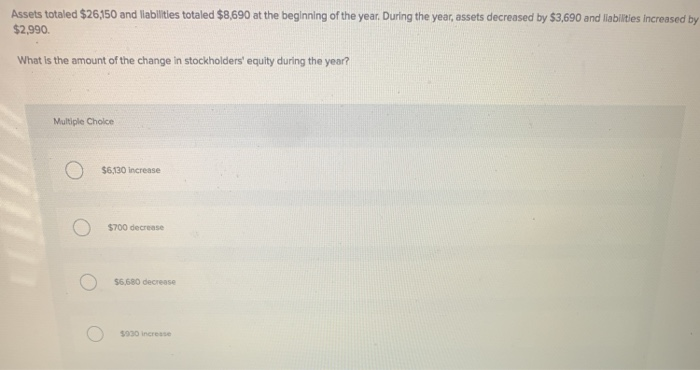Assets totaled \$26,150 and liabilities totaled \$8,690 at the beginning of the year. During the year, assets decreased by \$3,690 and liabilities increased by \$2,990. What is the amount of the change in stockholders' equity during the year? Multiple Choice Ο \$6,130 increase Ο \$700 decrease Ο \$6,680 decrease Ο \$930 increase

• ### Ending liabilities are 69,250, beginning equity was \$88,500, common stock issued during year totaled \$32,500, expenses...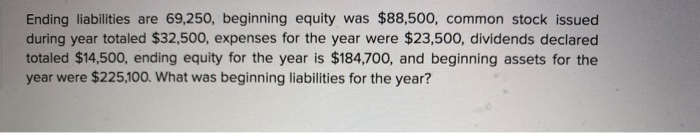Ending liabilities are 69,250, beginning equity was \$88,500, common stock issued during year totaled \$32,500, expenses for the year were \$23,500, dividends declared totaled \$14,500, ending equity for the year is \$184,700, and beginning assets for the year were \$225,100. What was beginning liabilities for the year? Multiple Choice \$217,200 \$155,850 \$136,600 \$157750 \$253,950 Return on assets is: Computed by multiplying net income by average total assets. Computed by dividing net income by average total assets. Used in helping evaluate...

• ### The Income Statement shows: . Multiple Choice o the change in owner's equity for a period...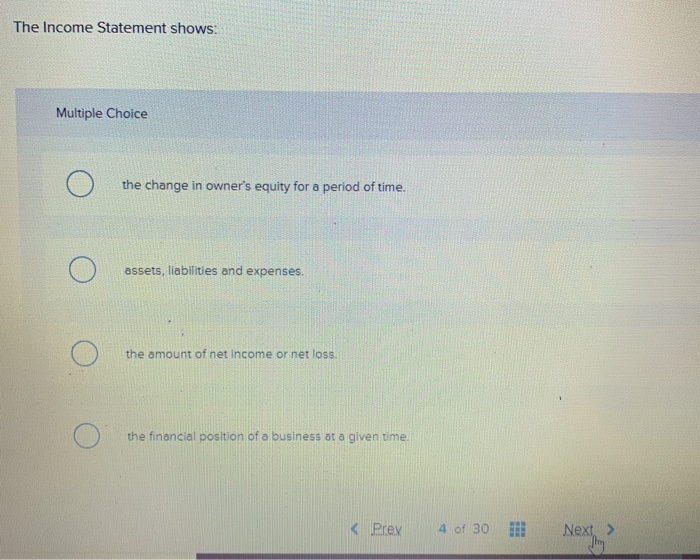The Income Statement shows: . Multiple Choice o the change in owner's equity for a period of time. o assets, liabilities and expenses. o the amount of net income or net loss. o the financial position of a business at a given time < Prey 4 of 30 !!! Next >

• ### The statement of cash flows reports: Multiple Choice points (3 02:19:16 Ο Revenues, gains, expenses, and...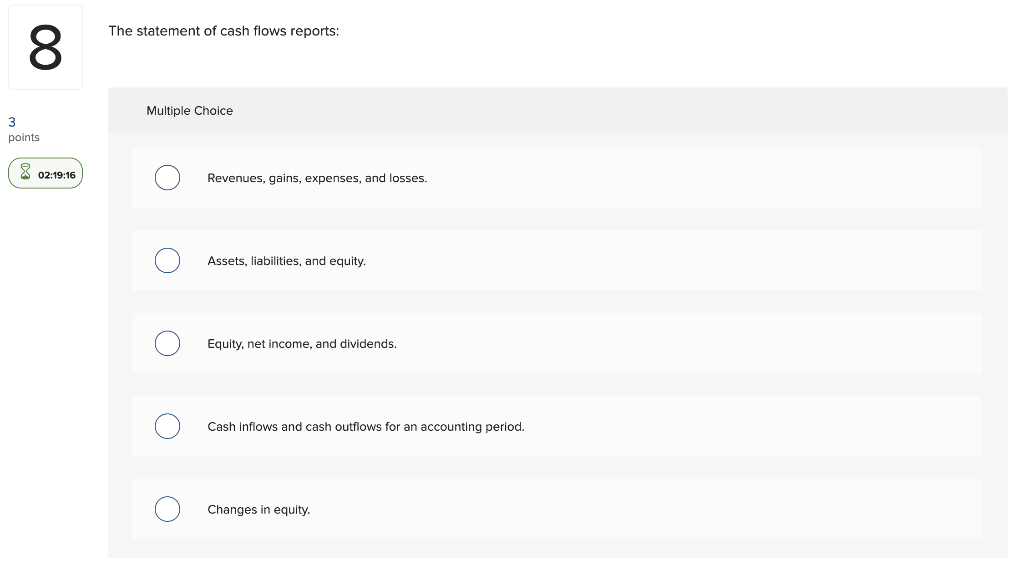The statement of cash flows reports: Multiple Choice points (3 02:19:16 Ο Revenues, gains, expenses, and losses. Ο Assets, liabilities, and equity. Ο Equity, net income, and dividends. Ο Cash inflows and cash outflows for an accounting period. Ο Changes in equity.

Free Homework App